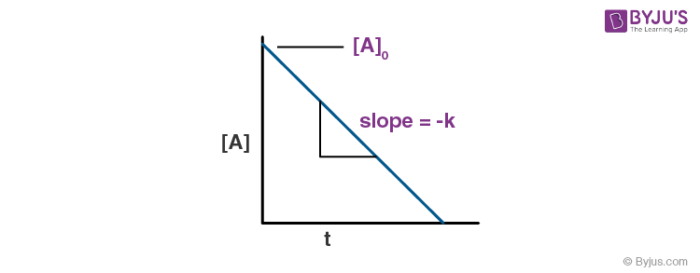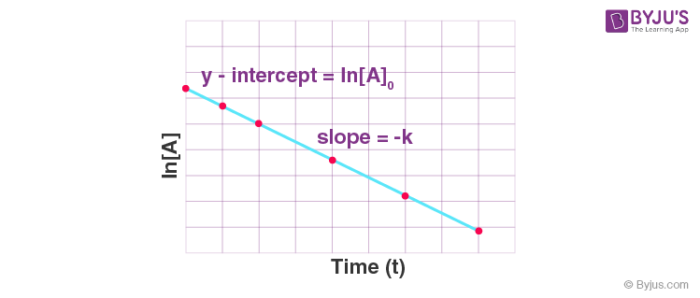# Integrated Rate Equation

An equation representing the dependence of the rate of reaction on the concentration of reacting species is known as a differential rate equation. The instantaneous rate of reaction is expressed as the slope of the tangent at any instant of time in the concentration-time graph. Hence, it is very difficult to determine the rate of reaction from the concentration-time graph. Therefore, we integrate the differential rate equation to obtain a relation between the concentration at different points and rate constant. This equation is known as the integrated rate equation. For reactions of different order, we observe different integrated rate equations.

Zero order reaction: In zero order reaction, the rate of reaction depends upon the zeroth power of concentration of reactants. Zero order reactions are very rarely observed. Some examples of zero order reactions are: thermal decomposition of HI on gold surface, decomposition of gaseous ammonia on a hot platinum surface etc. A general equation for a zero order reaction with rate constant k is derived below:

A → B

$$\begin{array}{l} Rate = – \frac {d[A]}{dt} =k[A]^\circ\end{array}$$
$$\begin{array}{l} -\frac {d[A]}{dt} = k\end{array}$$

=> d[A] = -k dt

Integrating both sides:

⇒ [A] = -kt + c………………………..(1)

Where, c= constant of integration,

At time, t=0,

$$\begin{array}{l} [A] = [A]_0\end{array}$$

Putting the limits in equation (1) we get the value of c,

$$\begin{array}{l} [A]_0 = c\end{array}$$

Using the value of c in equation (1) we get,

$$\begin{array}{l}[A] = -kt + [A]_0\end{array}$$

The above equation is known as the integrated rate equation for zero order reactions. We can observe the above equation as an equation of straight line with concentration of reactant on y-axis and time on x-axis. The slope of the straight line signifies the value of rate constant, k.First order reaction: In first order reaction, the rate of reaction depends upon the first power of concentration of reactants. Natural and artificial radioactive decay of unstable nuclei are examples of first order reactions. A general equation for a first order reaction with rate constant k is derived below:

A → B

$$\begin{array}{l}Rate = – \frac {d[A]}{dt} = k[A]\end{array}$$
$$\begin{array}{l}= \frac {d[A]}{[A]} = -k dt\end{array}$$

Integrating both sides:

=> ln [A] = -kt + c……………………….(2)

Where, c= constant of integration,

At time, t=0,

$$\begin{array}{l}[A] = [A]_0\end{array}$$

Putting the limits in equation (2) we get the value of c

$$\begin{array}{l} [A]_0 = c \end{array}$$

Using the value of c in the above equation, we get,

ln [A] = -kt + ln A0

We observe that the equation above can be plotted as a straight line with ln[A] on y-axis and time (t) on x-axis. The negative of the slope of this straight line gives us the value of the rate constant, k.We can also determine the value of the rate constant, k from the equation as:

$$\begin{array}{l} ln ~\frac {[A]}{[A]_0} = -kt\end{array}$$
$$\begin{array}{l}k = – \frac {ln~\frac {[A]}{[A]_0}}{t} \end{array}$$

Concentration at any moment of time can be given as,

$$\begin{array}{l}[A] = [A]_0 e^{-kt}\end{array}$$

Thus, we can determine the concentration and rate of reaction at any moment with the help of an integrated rate equation for zero and first order reaction.

Learn about the Use of Empirical and Molecular Formula in Chemistry. Download BYJU’S – The Learning App to master Chemistry.’

Test your Knowledge on Integrated rate equation!